Calculus Volume 1

# 5.1Approximating Areas

Calculus Volume 15.1 Approximating Areas

### Learning Objectives

• 5.1.1 Use sigma (summation) notation to calculate sums and powers of integers.
• 5.1.2 Use the sum of rectangular areas to approximate the area under a curve.
• 5.1.3 Use Riemann sums to approximate area.

Archimedes was fascinated with calculating the areas of various shapes—in other words, the amount of space enclosed by the shape. He used a process that has come to be known as the method of exhaustion, which used smaller and smaller shapes, the areas of which could be calculated exactly, to fill an irregular region and thereby obtain closer and closer approximations to the total area. In this process, an area bounded by curves is filled with rectangles, triangles, and shapes with exact area formulas. These areas are then summed to approximate the area of the curved region.

In this section, we develop techniques to approximate the area between a curve, defined by a function $f(x),f(x),$ and the x-axis on a closed interval $[a,b].[a,b].$ Like Archimedes, we first approximate the area under the curve using shapes of known area (namely, rectangles). By using smaller and smaller rectangles, we get closer and closer approximations to the area. Taking a limit allows us to calculate the exact area under the curve.

Let’s start by introducing some notation to make the calculations easier. We then consider the case when $f(x)f(x)$ is continuous and nonnegative. Later in the chapter, we relax some of these restrictions and develop techniques that apply in more general cases.

### Sigma (Summation) Notation

As mentioned, we will use shapes of known area to approximate the area of an irregular region bounded by curves. This process often requires adding up long strings of numbers. To make it easier to write down these lengthy sums, we look at some new notation here, called sigma notation (also known as summation notation). The Greek capital letter $Σ,Σ,$ sigma, is used to express long sums of values in a compact form. For example, if we want to add all the integers from 1 to 20 without sigma notation, we have to write

$1+2+3+4+5+6+7+8+9+10+11+12+13+14+15+16+17+18+19+20.1+2+3+4+5+6+7+8+9+10+11+12+13+14+15+16+17+18+19+20.$

We could probably skip writing a couple of terms and write

$1+2+3+4+⋯+19+20,1+2+3+4+⋯+19+20,$

which is better, but still cumbersome. With sigma notation, we write this sum as

$∑i=120i,∑i=120i,$

which is much more compact.

Typically, sigma notation is presented in the form

$∑i=1nai∑i=1nai$

where $aiai$ describes the terms to be added, and the i is called the index. Each term is evaluated, then we sum all the values, beginning with the value when $i=1i=1$ and ending with the value when $i=n.i=n.$ For example, an expression like $∑i=27si∑i=27si$ is interpreted as $s2+s3+s4+s5+s6+s7.s2+s3+s4+s5+s6+s7.$ Note that the index is used only to keep track of the terms to be added; it does not factor into the calculation of the sum itself. The index is therefore called a dummy variable. We can use any letter we like for the index. Typically, mathematicians use i, j, k, m, and n for indices.

Let’s try a couple of examples of using sigma notation.

### Example 5.1

#### Using Sigma Notation

1. Write in sigma notation and evaluate the sum of terms $3i3i$ for $i=1,2,3,4,5.i=1,2,3,4,5.$
2. Write the sum in sigma notation:
$1+14+19+116+125.1+14+19+116+125.$

### Checkpoint5.1

Write in sigma notation and evaluate the sum of terms 2i for $i=3,4,5,6.i=3,4,5,6.$

The properties associated with the summation process are given in the following rule.

### Rule: Properties of Sigma Notation

Let $a1,a2,…,ana1,a2,…,an$ and $b1,b2,…,bnb1,b2,…,bn$ represent two sequences of terms and let c be a constant. The following properties hold for all positive integers n and for integers m, with $1≤m≤n.1≤m≤n.$

1. $∑i=1nc=nc∑i=1nc=nc$
(5.1)

2. $∑i=1ncai=c∑i=1nai∑i=1ncai=c∑i=1nai$
(5.2)

3. $∑i=1n(ai+bi)=∑i=1nai+∑i=1nbi∑i=1n(ai+bi)=∑i=1nai+∑i=1nbi$
(5.3)

4. $∑i=1n(ai−bi)=∑i=1nai−∑i=1nbi∑i=1n(ai−bi)=∑i=1nai−∑i=1nbi$
(5.4)

5. $∑i=1nai=∑i=1mai+∑i=m+1nai∑i=1nai=∑i=1mai+∑i=m+1nai$
(5.5)

#### Proof

We prove properties 2. and 3. here, and leave proof of the other properties to the Exercises.

2. We have

$∑i=1ncai=ca1+ca2+ca3+⋯+can=c(a1+a2+a3+⋯+an)=c∑i=1nai.∑i=1ncai=ca1+ca2+ca3+⋯+can=c(a1+a2+a3+⋯+an)=c∑i=1nai.$

3. We have

$∑i=1n(ai+bi)=(a1+b1)+(a2+b2)+(a3+b3)+⋯+(an+bn)=(a1+a2+a3+⋯+an)+(b1+b2+b3+⋯+bn)=∑i=1nai+∑i=1nbi.∑i=1n(ai+bi)=(a1+b1)+(a2+b2)+(a3+b3)+⋯+(an+bn)=(a1+a2+a3+⋯+an)+(b1+b2+b3+⋯+bn)=∑i=1nai+∑i=1nbi.$

A few more formulas for frequently found functions simplify the summation process further. These are shown in the next rule, for sums and powers of integers, and we use them in the next set of examples.

### Rule: Sums and Powers of Integers

1. The sum of n integers is given by
$∑i=1ni=1+2+⋯+n=n(n+1)2.∑i=1ni=1+2+⋯+n=n(n+1)2.$
2. The sum of consecutive integers squared is given by
$∑i=1ni2=12+22+⋯+n2=n(n+1)(2n+1)6.∑i=1ni2=12+22+⋯+n2=n(n+1)(2n+1)6.$
3. The sum of consecutive integers cubed is given by
$∑i=1ni3=13+23+⋯+n3=n2(n+1)24.∑i=1ni3=13+23+⋯+n3=n2(n+1)24.$

### Example 5.2

#### Evaluation Using Sigma Notation

Write using sigma notation and evaluate:

1. The sum of the terms $(i−3)2(i−3)2$ for $i=1,2,…,200.i=1,2,…,200.$
2. The sum of the terms $(i3−i2)(i3−i2)$ for $i=1,2,3,4,5,6.i=1,2,3,4,5,6.$

### Checkpoint5.2

Find the sum of the values of $4+3i4+3i$ for $i=1,2,…,100.i=1,2,…,100.$

### Example 5.3

#### Finding the Sum of the Function Values

Find the sum of the values of $f(x)=x3f(x)=x3$ over the integers $1,2,3,…,10.1,2,3,…,10.$

### Checkpoint5.3

Evaluate the sum indicated by the notation $∑k=120(2k+1).∑k=120(2k+1).$

### Approximating Area

Now that we have the necessary notation, we return to the problem at hand: approximating the area under a curve. Let $f(x)f(x)$ be a continuous, nonnegative function defined on the closed interval $[a,b].[a,b].$ We want to approximate the area A bounded by $f(x)f(x)$ above, the x-axis below, the line $x=ax=a$ on the left, and the line $x=bx=b$ on the right (Figure 5.2).

Figure 5.2 An area (shaded region) bounded by the curve $f(x)f(x)$ at top, the x-axis at bottom, the line $x=ax=a$ to the left, and the line $x=bx=b$ at right.

How do we approximate the area under this curve? The approach is a geometric one. By dividing a region into many small shapes that have known area formulas, we can sum these areas and obtain a reasonable estimate of the true area. We begin by dividing the interval $[a,b][a,b]$ into n subintervals of equal width, $b−an.b−an.$ We do this by selecting equally spaced points $x0,x1,x2,…,xnx0,x1,x2,…,xn$ with $x0=a,xn=b,x0=a,xn=b,$ and

$xi−xi−1=b−anxi−xi−1=b−an$

for $i=1,2,3,…,n.i=1,2,3,…,n.$

We denote the width of each subinterval with the notation Δx, so $Δx=b−anΔx=b−an$ and

$xi=x0+iΔxxi=x0+iΔx$

for $i=1,2,3,…,n.i=1,2,3,…,n.$ This notion of dividing an interval $[a,b][a,b]$ into subintervals by selecting points from within the interval is used quite often in approximating the area under a curve, so let’s define some relevant terminology.

### Definition

A set of points $P={xi}P={xi}$ for $i=0,1,2,…,ni=0,1,2,…,n$ with $a=x0 which divides the interval $[a,b][a,b]$ into subintervals of the form $[x0,x1],[x1,x2],…,[xn−1,xn][x0,x1],[x1,x2],…,[xn−1,xn]$ is called a partition of $[a,b].[a,b].$ If the subintervals all have the same width, the set of points forms a regular partition of the interval $[a,b].[a,b].$

We can use this regular partition as the basis of a method for estimating the area under the curve. We next examine two methods: the left-endpoint approximation and the right-endpoint approximation.

### Rule: Left-Endpoint Approximation

On each subinterval $[xi−1,xi][xi−1,xi]$ (for $i=1,2,3,…,n),i=1,2,3,…,n),$ construct a rectangle with width Δx and height equal to $f(xi−1),f(xi−1),$ which is the function value at the left endpoint of the subinterval. Then the area of this rectangle is $f(xi−1)Δx.f(xi−1)Δx.$ Adding the areas of all these rectangles, we get an approximate value for A (Figure 5.3). We use the notation Ln to denote that this is a left-endpoint approximation of A using n subintervals.

$A≈Ln=f(x0)Δx+f(x1)Δx+⋯+f(xn−1)Δx=∑i=1nf(xi−1)ΔxA≈Ln=f(x0)Δx+f(x1)Δx+⋯+f(xn−1)Δx=∑i=1nf(xi−1)Δx$
(5.6)
Figure 5.3 In the left-endpoint approximation of area under a curve, the height of each rectangle is determined by the function value at the left of each subinterval.

The second method for approximating area under a curve is the right-endpoint approximation. It is almost the same as the left-endpoint approximation, but now the heights of the rectangles are determined by the function values at the right of each subinterval.

### Rule: Right-Endpoint Approximation

Construct a rectangle on each subinterval $[xi−1,xi],[xi−1,xi],$ only this time the height of the rectangle is determined by the function value $f(xi)f(xi)$ at the right endpoint of the subinterval. Then, the area of each rectangle is $f(xi)Δxf(xi)Δx$ and the approximation for A is given by

$A≈Rn=f(x1)Δx+f(x2)Δx+⋯+f(xn)Δx=∑i=1nf(xi)Δx.A≈Rn=f(x1)Δx+f(x2)Δx+⋯+f(xn)Δx=∑i=1nf(xi)Δx.$
(5.7)

The notation $RnRn$ indicates this is a right-endpoint approximation for A (Figure 5.4).

Figure 5.4 In the right-endpoint approximation of area under a curve, the height of each rectangle is determined by the function value at the right of each subinterval. Note that the right-endpoint approximation differs from the left-endpoint approximation in Figure 5.3.

The graphs in Figure 5.5 represent the curve $f(x)=x22.f(x)=x22.$ In graph (a) we divide the region represented by the interval $[0,3][0,3]$ into six subintervals, each of width 0.5. Thus, $Δx=0.5.Δx=0.5.$ We then form six rectangles by drawing vertical lines perpendicular to $xi−1,xi−1,$ the left endpoint of each subinterval. We determine the height of each rectangle by calculating $f(xi−1)f(xi−1)$ for $i=1,2,3,4,5,6.i=1,2,3,4,5,6.$ The intervals are $[0,0.5],[0.5,1],[1,1.5],[1.5,2],[2,2.5],[2.5,3].[0,0.5],[0.5,1],[1,1.5],[1.5,2],[2,2.5],[2.5,3].$ We find the area of each rectangle by multiplying the height by the width. Then, the sum of the rectangular areas approximates the area between $f(x)f(x)$ and the x-axis. When the left endpoints are used to calculate height, we have a left-endpoint approximation. Thus,

$A≈L6=∑i=16f(xi−1)Δx=f(x0)Δx+f(x1)Δx+f(x2)Δx+f(x3)Δx+f(x4)Δx+f(x5)Δx=f(0)0.5+f(0.5)0.5+f(1)0.5+f(1.5)0.5+f(2)0.5+f(2.5)0.5=(0)0.5+(0.125)0.5+(0.5)0.5+(1.125)0.5+(2)0.5+(3.125)0.5=0+0.0625+0.25+0.5625+1+1.5625=3.4375.A≈L6=∑i=16f(xi−1)Δx=f(x0)Δx+f(x1)Δx+f(x2)Δx+f(x3)Δx+f(x4)Δx+f(x5)Δx=f(0)0.5+f(0.5)0.5+f(1)0.5+f(1.5)0.5+f(2)0.5+f(2.5)0.5=(0)0.5+(0.125)0.5+(0.5)0.5+(1.125)0.5+(2)0.5+(3.125)0.5=0+0.0625+0.25+0.5625+1+1.5625=3.4375.$
Figure 5.5 Methods of approximating the area under a curve by using (a) the left endpoints and (b) the right endpoints.

In Figure 5.5(b), we draw vertical lines perpendicular to $xixi$ such that $xixi$ is the right endpoint of each subinterval, and calculate $f(xi)f(xi)$ for $i=1,2,3,4,5,6.i=1,2,3,4,5,6.$ We multiply each $f(xi)f(xi)$ by Δx to find the rectangular areas, and then add them. This is a right-endpoint approximation of the area under $f(x).f(x).$ Thus,

$A≈R6=∑i=16f(xi)Δx=f(x1)Δx+f(x2)Δx+f(x3)Δx+f(x4)Δx+f(x5)Δx+f(x6)Δx=f(0.5)0.5+f(1)0.5+f(1.5)0.5+f(2)0.5+f(2.5)0.5+f(3)0.5=(0.125)0.5+(0.5)0.5+(1.125)0.5+(2)0.5+(3.125)0.5+(4.5)0.5=0.0625+0.25+0.5625+1+1.5625+2.25=5.6875.A≈R6=∑i=16f(xi)Δx=f(x1)Δx+f(x2)Δx+f(x3)Δx+f(x4)Δx+f(x5)Δx+f(x6)Δx=f(0.5)0.5+f(1)0.5+f(1.5)0.5+f(2)0.5+f(2.5)0.5+f(3)0.5=(0.125)0.5+(0.5)0.5+(1.125)0.5+(2)0.5+(3.125)0.5+(4.5)0.5=0.0625+0.25+0.5625+1+1.5625+2.25=5.6875.$

### Example 5.4

#### Approximating the Area Under a Curve

Use both left-endpoint and right-endpoint approximations to approximate the area under the curve of $f(x)=x2f(x)=x2$ on the interval $[0,2];[0,2];$ use $n=4.n=4.$

### Checkpoint5.4

Sketch left-endpoint and right-endpoint approximations for $f(x)=1xf(x)=1x$ on $[1,2];[1,2];$ use $n=4.n=4.$ Approximate the area using both methods.

Looking at Figure 5.5 and the graphs in Example 5.4, we can see that when we use a small number of intervals, neither the left-endpoint approximation nor the right-endpoint approximation is a particularly accurate estimate of the area under the curve. However, it seems logical that if we increase the number of points in our partition, our estimate of A will improve. We will have more rectangles, but each rectangle will be thinner, so we will be able to fit the rectangles to the curve more precisely.

We can demonstrate the improved approximation obtained through smaller intervals with an example. Let’s explore the idea of increasing n, first in a left-endpoint approximation with four rectangles, then eight rectangles, and finally 32 rectangles. Then, let’s do the same thing in a right-endpoint approximation, using the same sets of intervals, of the same curved region. Figure 5.8 shows the area of the region under the curve $f(x)=(x−1)3+4f(x)=(x−1)3+4$ on the interval $[0,2][0,2]$ using a left-endpoint approximation where $n=4.n=4.$ The width of each rectangle is

$Δx=2−04=12.Δx=2−04=12.$

The area is approximated by the summed areas of the rectangles, or

$L4=f(0)(0.5)+f(0.5)(0.5)+f(1)(0.5)+f(1.5)0.5=7.5.L4=f(0)(0.5)+f(0.5)(0.5)+f(1)(0.5)+f(1.5)0.5=7.5.$
Figure 5.8 With a left-endpoint approximation and dividing the region from a to b into four equal intervals, the area under the curve is approximately equal to the sum of the areas of the rectangles.

Figure 5.9 shows the same curve divided into eight subintervals. Comparing the graph with four rectangles in Figure 5.8 with this graph with eight rectangles, we can see there appears to be less white space under the curve when $n=8.n=8.$ This white space is area under the curve we are unable to include using our approximation. The area of the rectangles is

$L8=f(0)(0.25)+f(0.25)(0.25)+f(0.5)(0.25)+f(0.75)(0.25)+f(1)(0.25)+f(1.25)(0.25)+f(1.5)(0.25)+f(1.75)(0.25)=7.75.L8=f(0)(0.25)+f(0.25)(0.25)+f(0.5)(0.25)+f(0.75)(0.25)+f(1)(0.25)+f(1.25)(0.25)+f(1.5)(0.25)+f(1.75)(0.25)=7.75.$
Figure 5.9 The region under the curve is divided into $n=8n=8$ rectangular areas of equal width for a left-endpoint approximation.

The graph in Figure 5.10 shows the same function with 32 rectangles inscribed under the curve. There appears to be little white space left. The area occupied by the rectangles is

$L32=f(0)(0.0625)+f(0.0625)(0.0625)+f(0.125)(0.0625)+⋯+f(1.9375)(0.0625)=7.9375.L32=f(0)(0.0625)+f(0.0625)(0.0625)+f(0.125)(0.0625)+⋯+f(1.9375)(0.0625)=7.9375.$
Figure 5.10 Here, 32 rectangles are inscribed under the curve for a left-endpoint approximation.

We can carry out a similar process for the right-endpoint approximation method. A right-endpoint approximation of the same curve, using four rectangles (Figure 5.11), yields an area

$R4=f(0.5)(0.5)+f(1)(0.5)+f(1.5)(0.5)+f(2)(0.5)=8.5.R4=f(0.5)(0.5)+f(1)(0.5)+f(1.5)(0.5)+f(2)(0.5)=8.5.$
Figure 5.11 Now we divide the area under the curve into four equal subintervals for a right-endpoint approximation.

Dividing the region over the interval $[0,2][0,2]$ into eight rectangles results in $Δx=2−08=0.25.Δx=2−08=0.25.$ The graph is shown in Figure 5.12. The area is

$R8=f(0.25)(0.25)+f(0.5)(0.25)+f(0.75)(0.25)+f(1)(0.25)+f(1.25)(0.25)+f(1.5)(0.25)+f(1.75)(0.25)+f(2)(0.25)=8.25.R8=f(0.25)(0.25)+f(0.5)(0.25)+f(0.75)(0.25)+f(1)(0.25)+f(1.25)(0.25)+f(1.5)(0.25)+f(1.75)(0.25)+f(2)(0.25)=8.25.$
Figure 5.12 Here we use right-endpoint approximation for a region divided into eight equal subintervals.

Last, the right-endpoint approximation with $n=32n=32$ is close to the actual area (Figure 5.13). The area is approximately

$R32=f(0.0625)(0.0625)+f(0.125)(0.0625)+f(0.1875)(0.0625)+⋯+f(2)(0.0625)=8.0625.R32=f(0.0625)(0.0625)+f(0.125)(0.0625)+f(0.1875)(0.0625)+⋯+f(2)(0.0625)=8.0625.$
Figure 5.13 The region is divided into 32 equal subintervals for a right-endpoint approximation.

Based on these figures and calculations, it appears we are on the right track; the rectangles appear to approximate the area under the curve better as n gets larger. Furthermore, as n increases, both the left-endpoint and right-endpoint approximations appear to approach an area of 8 square units. Table 5.1 shows a numerical comparison of the left- and right-endpoint methods. The idea that the approximations of the area under the curve get better and better as n gets larger and larger is very important, and we now explore this idea in more detail.

Values of n Approximate Area Ln Approximate Area Rn
$n=4n=4$ 7.5 8.5
$n=8n=8$ 7.75 8.25
$n=32n=32$ 7.94 8.06
Table 5.1 Converging Values of Left- and Right-Endpoint Approximations as n Increases

### Forming Riemann Sums

So far we have been using rectangles to approximate the area under a curve. The heights of these rectangles have been determined by evaluating the function at either the right or left endpoints of the subinterval $[xi−1,xi].[xi−1,xi].$ In reality, there is no reason to restrict evaluation of the function to one of these two points only. We could evaluate the function at any point $xi*xi*$ in the subinterval $[xi−1,xi],[xi−1,xi],$ and use $f(xi*)f(xi*)$ as the height of our rectangle. This gives us an estimate for the area of the form

$A≈∑i=1nf(xi*)Δx.A≈∑i=1nf(xi*)Δx.$

A sum of this form is called a Riemann sum, named for the 19th-century mathematician Bernhard Riemann, who developed the idea.

### Definition

Let $f(x)f(x)$ be defined on a closed interval $[a,b][a,b]$ and let P be a regular partition of $[a,b].[a,b].$ Let Δx be the width of each subinterval $[xi−1,xi][xi−1,xi]$ and for each i, let $xi*xi*$ be any point in $[xi−1,xi].[xi−1,xi].$ A Riemann sum is defined for $f(x)f(x)$ as

$∑i=1nf(xi*)Δx.∑i=1nf(xi*)Δx.$

Recall that with the left- and right-endpoint approximations, the estimates seem to get better and better as n get larger and larger. The same thing happens with Riemann sums. Riemann sums give better approximations for larger values of n. We are now ready to define the area under a curve in terms of Riemann sums.

### Definition

Let $f(x)f(x)$ be a continuous, nonnegative function on an interval $[a,b],[a,b],$ and let $∑i=1nf(xi*)Δx∑i=1nf(xi*)Δx$ be a Riemann sum for $f(x).f(x).$ Then, the area under the curve $y=f(x)y=f(x)$ on $[a,b][a,b]$ is given by

$A=limn→∞∑i=1nf(xi*)Δx.A=limn→∞∑i=1nf(xi*)Δx.$

### Media

See a graphical demonstration of the construction of a Riemann sum.

Some subtleties here are worth discussing. First, note that taking the limit of a sum is a little different from taking the limit of a function $f(x)f(x)$ as x goes to infinity. Limits of sums are discussed in detail in the chapter on Sequences and Series; however, for now we can assume that the computational techniques we used to compute limits of functions can also be used to calculate limits of sums.

Second, we must consider what to do if the expression converges to different limits for different choices of ${xi*}.{xi*}.$ Fortunately, this does not happen. Although the proof is beyond the scope of this text, it can be shown that if $f(x)f(x)$ is continuous on the closed interval $[a,b],[a,b],$ then $limn→∞∑i=1nf(xi*)Δxlimn→∞∑i=1nf(xi*)Δx$ exists and is unique (in other words, it does not depend on the choice of ${xi*}).{xi*}).$

We look at some examples shortly. But, before we do, let’s take a moment and talk about some specific choices for ${xi*}.{xi*}.$ Although any choice for ${xi*}{xi*}$ gives us an estimate of the area under the curve, we don’t necessarily know whether that estimate is too high (overestimate) or too low (underestimate). If it is important to know whether our estimate is high or low, we can select our value for ${xi*}{xi*}$ to guarantee one result or the other.

If we want an overestimate, for example, we can choose ${xi*}{xi*}$ such that for $i=1,2,3,…,n,f(xi*)≥f(x)i=1,2,3,…,n,f(xi*)≥f(x)$ for all $x∈[xi−1,xi].x∈[xi−1,xi].$ In other words, we choose ${xi*}{xi*}$ so that for $i=1,2,3,…,n,f(xi*)i=1,2,3,…,n,f(xi*)$ is the maximum function value on the interval $[xi−1,xi].[xi−1,xi].$ If we select ${xi*}{xi*}$ in this way, then the Riemann sum $∑i=1nf(xi*)Δx∑i=1nf(xi*)Δx$ is called an upper sum. Similarly, if we want an underestimate, we can choose ${xi*}{xi*}$ so that for $i=1,2,3,…,n,f(xi*)i=1,2,3,…,n,f(xi*)$ is the minimum function value on the interval $[xi−1,xi].[xi−1,xi].$ In this case, the associated Riemann sum is called a lower sum. Note that if $f(x)f(x)$ is either increasing or decreasing throughout the interval $[a,b],[a,b],$ then the maximum and minimum values of the function occur at the endpoints of the subintervals, so the upper and lower sums are just the same as the left- and right-endpoint approximations.

### Example 5.5

#### Finding Lower and Upper Sums

Find a lower sum for $f(x)=10−x2f(x)=10−x2$ on $[1,2];[1,2];$ let $n=4n=4$ subintervals.

### Checkpoint5.5

1. Find an upper sum for $f(x)=10−x2f(x)=10−x2$ on $[1,2];[1,2];$ let $n=4.n=4.$
2. Sketch the approximation.

### Example 5.6

#### Finding Lower and Upper Sums for $f(x)=sinxf(x)=sinx$

Find a lower sum for $f(x)=sinxf(x)=sinx$ over the interval $[a,b]=[0,π2];[a,b]=[0,π2];$ let $n=6.n=6.$

### Checkpoint5.6

Using the function $f(x)=sinxf(x)=sinx$ over the interval $[0,π2],[0,π2],$ find an upper sum; let $n=6.n=6.$

### Section 5.1 Exercises

1.

State whether the given sums are equal or unequal.

1. $∑i=110i∑i=110i$ and $∑k=110k∑k=110k$
2. $∑i=110i∑i=110i$ and $∑i=615(i−5)∑i=615(i−5)$
3. $∑i=110i(i−1)∑i=110i(i−1)$ and $∑j=09(j+1)j∑j=09(j+1)j$
4. $∑i=110i(i−1)∑i=110i(i−1)$ and $∑k=110(k2−k)∑k=110(k2−k)$

In the following exercises, use the rules for sums of powers of integers to compute the sums.

2.

$∑ i = 5 10 i ∑ i = 5 10 i$

3.

$∑ i = 5 10 i 2 ∑ i = 5 10 i 2$

Suppose that $∑i=1100ai=15∑i=1100ai=15$ and $∑i=1100bi=−12.∑i=1100bi=−12.$ In the following exercises, compute the sums.

4.

$∑ i = 1 100 ( a i + b i ) ∑ i = 1 100 ( a i + b i )$

5.

$∑ i = 1 100 ( a i − b i ) ∑ i = 1 100 ( a i − b i )$

6.

$∑ i = 1 100 ( 3 a i − 4 b i ) ∑ i = 1 100 ( 3 a i − 4 b i )$

7.

$∑ i = 1 100 ( 5 a i + 4 b i ) ∑ i = 1 100 ( 5 a i + 4 b i )$

In the following exercises, use summation properties and formulas to rewrite and evaluate the sums.

8.

$∑ k = 1 20 100 ( k 2 − 5 k + 1 ) ∑ k = 1 20 100 ( k 2 − 5 k + 1 )$

9.

$∑ j = 1 50 ( j 2 − 2 j ) ∑ j = 1 50 ( j 2 − 2 j )$

10.

$∑ j = 11 20 ( j 2 − 10 j ) ∑ j = 11 20 ( j 2 − 10 j )$

11.

$∑ k = 1 25 [ ( 2 k ) 2 − 100 k ] ∑ k = 1 25 [ ( 2 k ) 2 − 100 k ]$

Let $LnLn$ denote the left-endpoint sum using n subintervals and let $RnRn$ denote the corresponding right-endpoint sum. In the following exercises, compute the indicated left and right sums for the given functions on the indicated interval.

12.

L4 for $f(x)=1x−1f(x)=1x−1$ on $[2,3][2,3]$

13.

R4 for $g(x)=cos(πx)g(x)=cos(πx)$ on $[0,1][0,1]$

14.

L6 for $f(x)=1x(x−1)f(x)=1x(x−1)$ on $[2,5][2,5]$

15.

R6 for $f(x)=1x(x−1)f(x)=1x(x−1)$ on $[2,5][2,5]$

16.

R4 for $1x2+11x2+1$ on $[−2,2][−2,2]$

17.

L4 for $1x2+11x2+1$ on $[−2,2][−2,2]$

18.

R4 for $x2−2x+1x2−2x+1$ on $[0,2][0,2]$

19.

L8 for $x2−2x+1x2−2x+1$ on $[0,2][0,2]$

20.

Compute the left and right Riemann sums—L4 and R4, respectively—for $f(x)=(2−|x|)f(x)=(2−|x|)$ on $[−2,2].[−2,2].$ Compute their average value and compare it with the area under the graph of f.

21.

Compute the left and right Riemann sums—L6 and R6, respectively—for $f(x)=(3−|3−x|)f(x)=(3−|3−x|)$ on $[0,6].[0,6].$ Compute their average value and compare it with the area under the graph of f.

22.

Compute the left and right Riemann sums—L4 and R4, respectively—for $f(x)=4−x2f(x)=4−x2$ on $[−2,2][−2,2]$ and compare their values.

23.

Compute the left and right Riemann sums—L6 and R6, respectively—for $f(x)=9−(x−3)2f(x)=9−(x−3)2$ on $[0,6][0,6]$ and compare their values.

Express the following endpoint sums in sigma notation but do not evaluate them.

24.

L30 for $f(x)=x2f(x)=x2$ on $[1,2][1,2]$

25.

L10 for $f(x)=4−x2f(x)=4−x2$ on $[−2,2][−2,2]$

26.

R20 for $f(x)=sinxf(x)=sinx$ on $[0,π][0,π]$

27.

R100 for $lnxlnx$ on $[1,e][1,e]$

In the following exercises, graph the function then use a calculator or a computer program to evaluate the following left and right endpoint sums. Is the area under the curve on the given interval better approximated by the left Riemann sum or right Riemann sum? If the two agree, say "neither."

28.

[T] L100 and R100 for $y=x2−3x+1y=x2−3x+1$ on the interval $[−1,1][−1,1]$

29.

[T] L100 and R100 for $y=x2y=x2$ on the interval $[0,1][0,1]$

30.

[T] L50 and R50 for $y=x+1x2−1y=x+1x2−1$ on the interval $[2,4][2,4]$

31.

[T] L100 and R100 for $y=x3y=x3$ on the interval $[−1,1][−1,1]$

32.

[T] L50 and R50 for $y=tan(x)y=tan(x)$ on the interval $[0,π4][0,π4]$

33.

[T] L100 and R100 for $y=e2xy=e2x$ on the interval $[−1,1][−1,1]$

34.

Let tj denote the time that it took Tejay van Garteren to ride the jth stage of the Tour de France in 2014. If there were a total of 21 stages, interpret $∑j=121tj.∑j=121tj.$

35.

Let $rjrj$ denote the total rainfall in Portland on the jth day of the year in 2009. Interpret $∑j=131rj.∑j=131rj.$

36.

Let $djdj$ denote the hours of daylight and $δjδj$ denote the increase in the hours of daylight from day $j−1j−1$ to day j in Fargo, North Dakota, on the jth day of the year. Interpret $d1+∑j=2365δj.d1+∑j=2365δj.$

37.

To help get in shape, Joe gets a new pair of running shoes. If Joe runs 1 mi each day in week 1 and adds $110110$ mi to his daily routine each week, what is the total mileage on Joe’s shoes after 25 weeks?

38.

The following table gives approximate values of the average annual atmospheric rate of increase in carbon dioxide (CO2) each decade since 1960, in parts per million (ppm). Estimate the total increase in atmospheric CO2 between 1964 and 2013.

1964–1973 1.07
1974–1983 1.34
1984–1993 1.40
1994–2003 1.87
2004–2013 2.07
Table 5.2 Average Annual Atmospheric CO2 Increase, 1964–2013 Source: http://www.esrl.noaa.gov/gmd/ccgg/trends/.
39.

The following table gives the approximate increase in sea level in inches over 20 years starting in the given year. Estimate the net change in mean sea level from 1870 to 2010.

Starting Year 20-Year Change
1870 0.3
1890 1.5
1910 0.2
1930 2.8
1950 0.7
1970 1.1
1990 1.5
Table 5.3 Approximate 20-Year Sea Level Increases, 1870–1990 Source: http://link.springer.com/article/10.1007%2Fs10712-011-9119-1
40.

The following table gives the approximate increase in dollars in the average price of a gallon of gas per decade since 1950. If the average price of a gallon of gas in 2010 was \$2.60, what was the average price of a gallon of gas in 1950?

Starting Year 10-Year Change
1950 0.03
1960 0.05
1970 0.86
1980 −0.03
1990 0.29
2000 1.12
Table 5.4 Approximate 10-Year Gas Price Increases, 1950–2000 Source: http://epb.lbl.gov/homepages/Rick_Diamond/docs/lbnl55011-trends.pdf.
41.

The following table gives the percent growth of the U.S. population beginning in July of the year indicated. If the U.S. population was 281,421,906 in July 2000, estimate the U.S. population in July 2010.

Year % Change/Year
2000 1.12
2001 0.99
2002 0.93
2003 0.86
2004 0.93
2005 0.93
2006 0.97
2007 0.96
2008 0.95
2009 0.88
Table 5.5 Annual Percentage Growth of U.S. Population, 2000–2009 Source: http://www.census.gov/popest/data.

(Hint: To obtain the population in July 2001, multiply the population in July 2000 by 1.0112 to get 284,573,831.)

In the following exercises, estimate the areas under the curves by computing the left Riemann sums, L8.

42.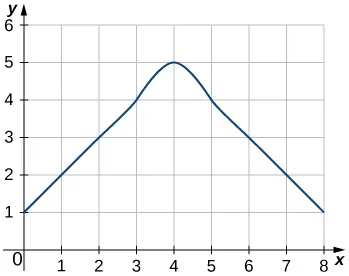43.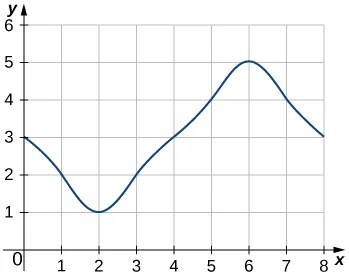44.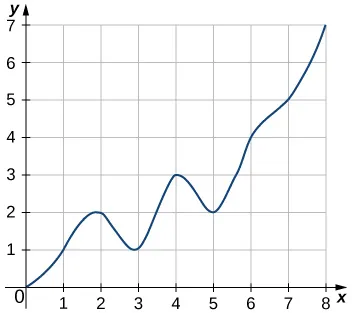45.46.

[T] Use a computer algebra system to compute the Riemann sum, $LN,LN,$ for $N=10,30,50N=10,30,50$ for $f(x)=1−x2f(x)=1−x2$ on $[−1,1].[−1,1].$

47.

[T] Use a computer algebra system to compute the Riemann sum, LN, for $N=10,30,50N=10,30,50$ for $f(x)=11+x2f(x)=11+x2$ on $[−1,1].[−1,1].$

48.

[T] Use a computer algebra system to compute the Riemann sum, LN, for $N=10,30,50N=10,30,50$ for $f(x)=sin2xf(x)=sin2x$ on $[0,2π].[0,2π].$ Compare these estimates with π.

In the following exercises, use a calculator or a computer program to evaluate the endpoint sums RN and LN for $N=1,10,100.N=1,10,100.$ How do these estimates compare with the exact answers, which you can find via geometry?

49.

[T] $y=cos(πx)y=cos(πx)$ on the interval $[0,1][0,1]$

50.

[T] $y=3x+2y=3x+2$ on the interval $[3,5][3,5]$

In the following exercises, use a calculator or a computer program to evaluate the endpoint sums RN and LN for $N=1,10,100.N=1,10,100.$

51.

[T] $y=x4−5x2+4y=x4−5x2+4$ on the interval $[−2,2],[−2,2],$ which has an exact area of $32153215$

52.

[T] $y=lnxy=lnx$ on the interval $[1,2],[1,2],$ which has an exact area of $2ln(2)−12ln(2)−1$

53.

Explain why, if $f(a)≥0f(a)≥0$ and f is increasing on $[a,b],[a,b],$ that the left endpoint estimate is a lower bound for the area below the graph of f on $[a,b].[a,b].$

54.

Explain why, if $f(b)≥0f(b)≥0$ and f is decreasing on $[a,b],[a,b],$ that the left endpoint estimate is an upper bound for the area below the graph of f on $[a,b].[a,b].$

55.

Show that, in general, $RN−LN=(b−a)×f(b)−f(a)N.RN−LN=(b−a)×f(b)−f(a)N.$

56.

Explain why, if f is increasing on $[a,b],[a,b],$ the error between either LN or RN and the area A below the graph of f is at most $(b−a)f(b)−f(a)N.(b−a)f(b)−f(a)N.$

57.

For each of the three graphs:

1. Obtain a lower bound $L(A)L(A)$ for the area enclosed by the curve by adding the areas of the squares enclosed completely by the curve.
2. Obtain an upper bound $U(A)U(A)$ for the area by adding to $L(A)L(A)$ the areas $B(A)B(A)$ of the squares enclosed partially by the curve.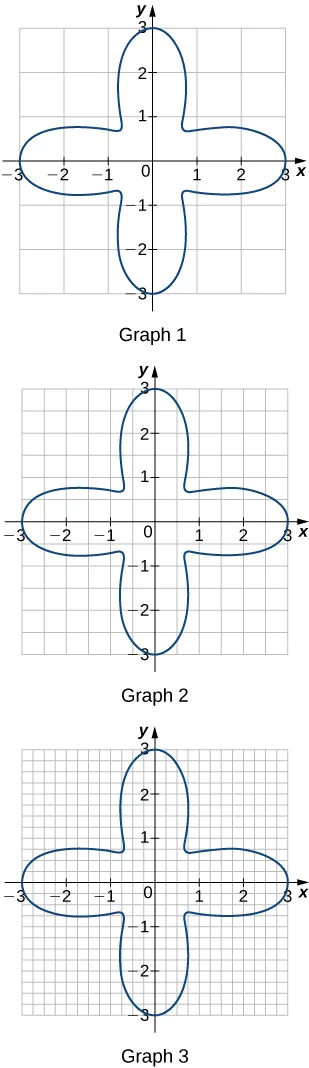58.

In the previous exercise, explain why $L(A)L(A)$ gets no smaller while $U(A)U(A)$ gets no larger as the squares are subdivided into four boxes of equal area.

59.

A unit circle is made up of n wedges equivalent to the inner wedge in the figure. The base of the inner triangle is 1 unit and its height is $sin( 2πn ).sin( 2πn ).$ The base of the outer triangle is $B=cos( πn )+sin( πn )tan( πn )B=cos( πn )+sin( πn )tan( πn )$ and the height is $H=Bsin( 2πn).H=Bsin( 2πn).$ Use this information to argue that the area of a unit circle is equal to π.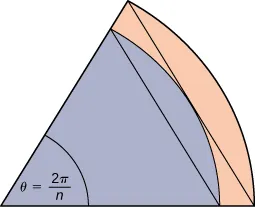Order a print copy

As an Amazon Associate we earn from qualifying purchases.